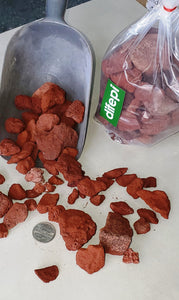ORDER A SAMPLE BEFORE YOU BUY
ORDER A SAMPLE BEFORE YOU BUY# Volcanic Lava Rock (Red)

Regular price \$29.00 \$0.00 Unit price per
Tax included.
Natural lava rocks
These rocks are durable, long-lasting, and have a fascinating burning effect.
Resist the most extreme temperatures without damaging or crumbling, add realism or create depth to your gas logs.
Perfect for fireplaces, bonfires, indoor or outdoor fireplaces, liquid propane or natural gas. Also can be used decoratively.
Calculate how many lava rocks you need, round / round bonfire, to calculate how many pounds of lava rocks you will need for your project, simply: radius (inches) x radius (inches) x 3.14 inches = square inches, then divide the square inches you have times 16 = number of pounds you will need (this is calculated for the optimal depth for lava rocks 2 inches deep.)
Calculate how much lava rocks you need - square / rectangular bonfire - Width (left and right) x Depth (front to back) = square inches / then divide the square inches you have by 16 = Number of pounds you will need to get how many pounds of lava rocks you will need (this is calculated for the optimal depth for 2 inch deep lava rocks).